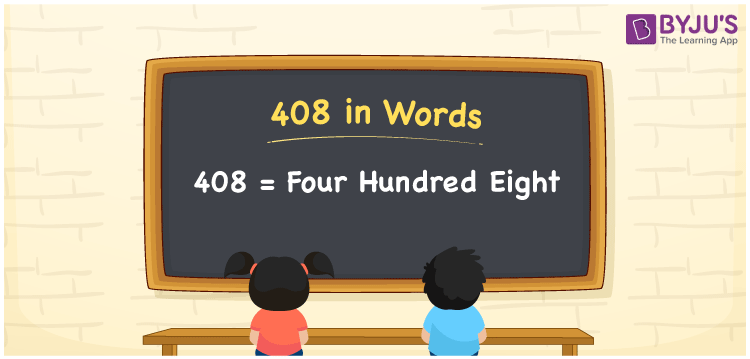# 408 in Words

408 in words is written as Four Hundred Eight. The name of number 408 in English is “Four Hundred Eight”. The word Four Hundred Eight is used in many real-life situations. For example, there are Four Hundred Eight students in a classroom. Thus, it is a counting number. Also, to represent the currency equal to 408, we can write it in words as Rupees Four Hundred Eight or Four Hundred Eight rupees. Therefore, it is necessary to learn the numbers in words, for the ease of understanding and expressing them.

 408 in words Four Hundred Eight Four Hundred Eight in Numbers 408

## 408 in English Words## How to Write 408 in Words?

If we know the place value of digits of 408, then we can easily express it in words. The place value is basically the position of a digit in a number. 408 is a three-digit number, therefore, we can specify the position of each digit of 408 in a place value chart. In the Indian numbering system, the order of place value of digits from right to left is given by:

 Hundreds Tens Ones 4 0 8

We can write it in expanded form as:

4 × Hundred + 0 × Ten + 8 × One

= 4 × 100 + 0 × 10 + 8 × 1

= 400 + 00 + 8

= 408

= Four Hundred Eight

Therefore, 408 in words is written as Four hundred and Eight.

408 is a natural number that precedes 409 and succeeds 407.

408 in words – Four Hundred Eight

Is 408 an odd number? – No

Is 408 an even number? – Yes

Is 408 a perfect square number? – No

Is 408 a perfect cube number? – No

Is 408 a prime number? – No

Is 408 a composite number? – Yes

## Frequently Asked Questions on 408 in Words

Q1

### How to write 408 in words?

408 in English is written as “Four Hundred Eight”.
Q2

### Is 408 an odd number or even number?

408 is an even number because it is wholly divisible by 2.
Q3

### Write Four Hundred Eight in numbers.

Four Hundred Eight in numbers is 408.